• +91 9971497814
• info@interviewmaterial.com

# RD Chapter 1- Real Numbers Ex-1.2 Interview Questions Answers

### Related Subjects

Question 1 :
Define H.C.F. of two positive integers and find the H.C.F. of the following pairs of numbers.
(i) 32 and 54
(ii) 18 and 24
(iii) 70 and 30
(iv) 56 and 88
(v) 475 and 495
(vi) 75 and 243
(vii) 240 and 6552
(viii) 155 and 1385
(ix) 100 and 190
(x) 105 and 120

Answer 1 : Definition : The greatest among the common divisor oftwo or more integers is the Greatest Common Divisor (G.C.D.) or Highest CommonFactor (H.C.F.) of the given integers.
(i) HC.F. of 32 and 54
Factors 32 = 1, 2, 4, 8, 16, 32
and factors of 54 = 1, 2, 3, 6, 9, 18, 27, 54
H.C.F. = 2

(ii) H.C.F. of 18 and 24
Factors of 18 = 1, 2, 3, 6, 9, 18
and factors of 24 = 1, 2, 3, 4, 6, 8, 12, 24
Highest common factor = 6
H.C.F. = 6

(iii) H.C.F. of 70 and 30
Factors of 70 = 1, 2, 5, 7, 10, 14, 35, 70
and factors of 30 = 1, 2, 3, 5, 6, 10, 15, 30
H.C.F. = 10

(iv) H.C.F. of 56 and 88
Factors of 56 = 1, 2, 4, 7, 8, 14, 28, 56
and factors of 88 = 1, 2, 4, 8, 11, 22, 44, 88
H.C.F. = 8

(v) H.C.F. of 475 and 495
Factors of 475 = 1, 5, 25, 19, 95, 475
and factors of 495 = 1, 3, 5, 9, 11, 15, 33, 45, 55, 99, 165, 495
H.C.F. = 5

(vi) H.C.F. of 75 and 243
Factors of 75 = 1, 3, 5, 15, 25, 75
Factors of 243 = 1, 3, 9, 27, 81, 243
H.C.F. = 3

(vii) H.C.F. of 240 and 6552
Factors of 240 = 1, 2, 3, 4, 5, 6, 8, 10, 12, 15, 16, 20, 24, 48, 60, 80, 120,240
Factors of 6552 = 1, 2, 3, 4, 6, 7, 8, 9, 12, 13, 14, 18, 21, 24, 26, 28, 36,39, 42, 52, 56, 63, 72, 91, 104, 117, 126, 156, 168, 182, 234,252, 273, 312,364, 488, 504, 546, 728, 819, 936, 1092, 1638, 2184, 3276, 6552
H.C.F. = 24

(viii) H.C.F. of 155 and 1385
Factors of 155 = 1, 5, 31, 155
Factors of 1385 = 1, 5, 277, 1385
H.C.F. = 5

(ix) 100 and 190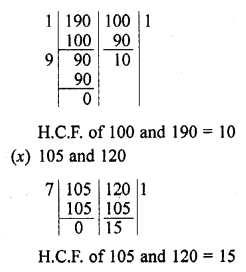Question 2 :
Use Euclid’s division algorithm to find the H.C.F. of
(i) 135 and 225
(ii) 196 and 38220
(iii) 867 and 255
(iv) 184, 230 and 276
(v) 136,170 and 255

(i) H.C.F. of 135 and 225
135 < 225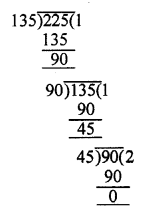225 = 135 x 1 + 90
135 = 90 x 1 +45
45 = 45 x 2 + 0
Last remainder = 0
and last divisor = 45
H.C.F. = 45
(ii) H.C.F. of 196 and 38220
196 < 38220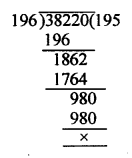38220 = 196 x 195 + 0
Last remainder = 0
and last divisor = 196
H.C.F. = 196
(iii) H.C.F. 867 and 255
255 < 867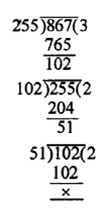867 = 255 x 3 + 102
255 = 102 x 2 + 51
102 = 51 x 2 + 0
Last remainder = 0
and last divisor = 51
H.C.F. = 51
(iv) H.C.F. of 184, 230 and 276
Let us find the highest common factor (H.C.F.) of 184 and 230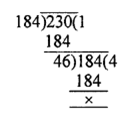Hence, H.C.F. of 184 and 230 = 46
Now, find the H.C.F. of 276 and 46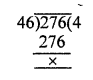Hence, H.C.F. of 276 and 46 = 46
Required H.C.F. of 184, 230 and 276 = 46
(v) H.C.F. of 136, 170 and 255
Let us find the highest common factor (H.C.F.) of 136 and 70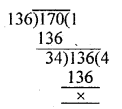Hence, H.C.F. of 136 and 170 = 34
Now, find the H.C.F. of 34 and 255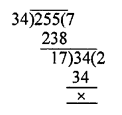Hence, highest common factor of 34 and 255 = 17
Required H.C.F. of 136, 170 and 255 = 17

Question 3 :
Find the H.C.F. of the following pairs of integers and express it as a linear combinations of them.
(i) 963 and 657
(ii) 592 and 252
(iii) 506 and 1155
(iv) 1288 and 575

(i) 963 and 657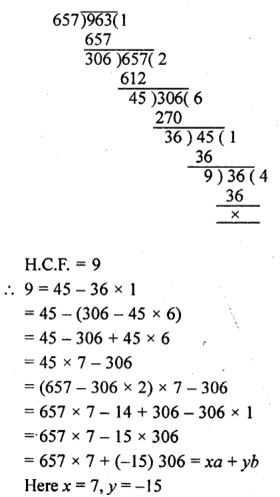(ii) HCF of 592 and 252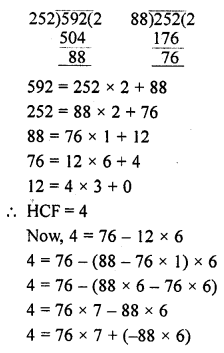76x + 88y
Where x = 7, y = -6
(iii) 506 and 1155
H.C.F. = 11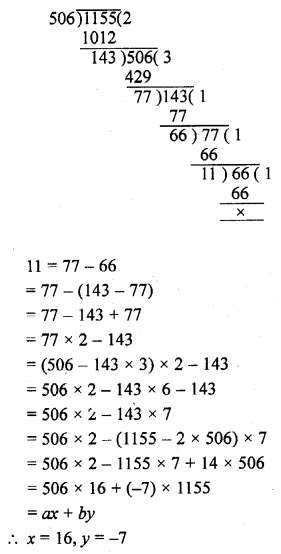(iv) 1288 and 575
H.C.F. = 23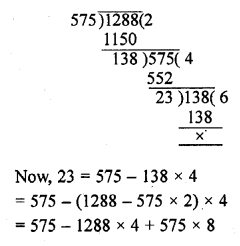= 575 x 9 + 1288 x (-4)
= ax + by
x = 9, y = -4

Question 4 : Find the largest number which divides 615 and 963 leaving remainder 6 in each case.

The given numbers are 615 and 963
Remainder in each case = 6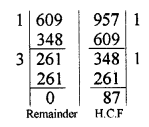615 – 6 = 609 and 963 – 6 = 957 are divisible by the required number which is the H.C.F. of 609 and 957 = 87
Hence the required largest number = 87

Question 5 : If the H.C.F. of 408 and 1032 is expressible in the form 1032m – 408 x 5, find m.

408, 1032
H.C.F. = 24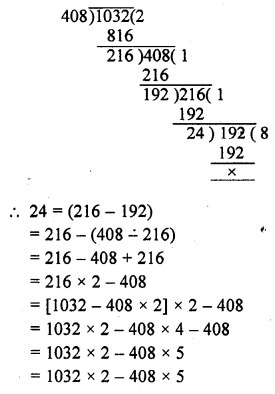Which is in the form of 1032m – 408 x 5 comparing, we get m = 2

Question 6 : If the H.C.F. of 657 and 963 is expressible in the form 657x + 963 x (-15), find x.

657 and 963
H.C.F. = 9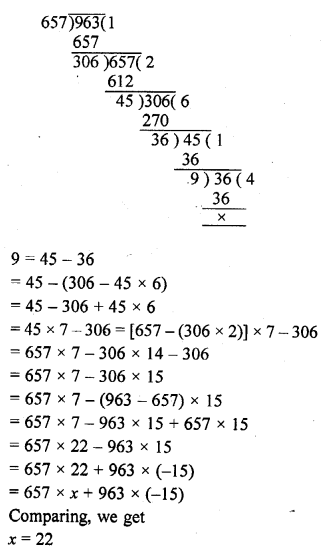Question 7 : An army contingent of 616 members is to march behind an army band of 32 members in a parade. The two groups are to march in the same number of columns. What is the maximum number of columns in which they can march ?

The required number of columns will be the H.C.F. of 616 and 32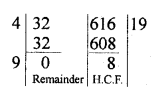Using Euclid’s division
We get H.C.F. = 4
Number of columns = 4

Question 8 : A merchant has 120 litres of oil of one kind, 180 litres of another kind and 240 litres of third kind. He wants to sell the oil by filling the three kinds of oil in tins of equal capacity. What should be the greatest capacity of such a tin ?

Quantity of oil of one kind =120l
and quantity of second kind = 180l
and third kind of oil = 240l
Maximum capacity of oil in each tin = H.C.F. of 120l, 180l and 240l
H.C.F. of 120 and 180 = 60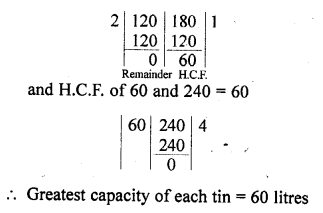Question 9 : During a sale, colour pencils were being sold in packs of 24 each and crayons in packs of 32 each. If you want full packs of both and the same number of pencils and crayons, how many of each would you need to buy ?

Number of pencils in each pack = 24
and number of crayons pack = 32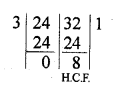Highest number of pencils and crayons in packs will be = H.C.F. of 24 and 32 = 8
Number of pencil’s pack = 24/8 = 3
and number of crayon’s pack = 32/8 = 4

Question 10 : 144 cartons of Coke Cans and 90 Cartons of Pepsi Cans, are to be stacked in a Canteen. If each stack is of the same height and is to contain cartons of the same drink what would be the greatest number of cartons each stack would have?

Number of Coke Cans Cartons = 144
and number Pepsi Cartons = 90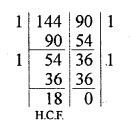Required greatest number of cartons of each = H.C.F. of 144 and 90 = 18

Todays Deals### RD Chapter 1- Real Numbers Ex-1.2 Contributorskrishan

Name:
Email:

# Latest News# 9000 interview questions in different categories